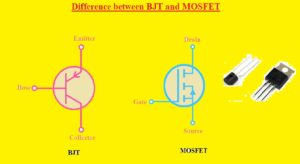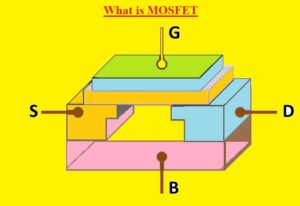Hello, readers welcome to the new post. We will discuss Difference between BJT and MOSFET. These two are electronic components and belongs to the transistor family. But used for different applications on the basis of different structures.

In this post, we will discuss different parameters to find the differences between these two transistors. So let’s get started

### What is BJT

• BJT stands for bipolar junction transistors and its structure consists of two PN junctions. It has two types NPN and PNP.
• There are 3 pins that have these components emitter-base and collector.
• In the case of NPN transistors, this component as P layers positioned between the two N regions. while in the case of PNP N layer is positioned between two P layers.
• Its common applications are amplifier circuits switching and oscillators. It knew as current controlled components that used bases current to regulate the emitter and collector current. The collector is larger parts and has large value of current.
• The small value of signal given at the base pin of transistors will get in amplified at the collector. There is no need of dc power supply to perform the amplification
• There are two charge carriers that help to flow current in the BJT electrons and holes.
• The larger value current is collectors that is regulated through the base current IB and this feature helps to use for switches and amplifier circuits.
• BJT is known as the current controlled module### MOSFET

• MOSFET stands for metal oxide semiconductor field effect transistor created through the use of semiconductor material.
• It commonly used transistors have 3 pinouts that are gate source and drain.
• It comes with metallic structure at the upper part of silicon oxide layers below with semiconductors.
• There are two types of these transistors E MOSFET and D MOSFET.
• E MOSFET remains off in normal operating conditions and needs gate volts to get on where the depletion MOSFET is on during normal conditions need gate volts to get off
• In 1959, MOSFET was first created by Egyptian engineer Mohamed M.Atalla and Dawon Kahng who was Korian engineer.
• This electronic component is most commonly used in electronic circuits, and nearly  (1.3 × 1022) MOSFETs have been created from 1960 to 2018.
• It is also very often used in digital, analog circuits and power devices.
• The main advantages of MOSFETs are that no input current is needed to regulate the load current as required in a BJT.### BJT vs MOSFET• The main types of BJT are NPN and PNP while types of MOSFET are E MOSFET and D MOSFET
• BJT pins are emitter-base and collector and MOSFET pins are gate source and drain
• BJT is bipolar and MOSFET is unipolar transistor
• For BJT electrons and holes work as charge carries and for MOSFET anyone can work as a charge carrier
• BJT is used for current control applications and MOSFET is used for voltage control circuits
• Switching speed of BJT is one hundred kilohertz and MOSFET has 300KHZ
• The value of input impedance for BJT is less than MOSFET
• Output impedance for BJT is low and MOSFET has a medium level
• BJT has negative temperatures coefficient not used for parallel operations nd MOSFET is used for parallel operations since have a positive temperatures coefficient
• MOSFET uses less power than the BJT

That is all about the Difference between BJT and MOSFET all details has explained to find the differences between BJT and MOSFET. If you have any queries ask in the comments. Thanks for reading have a nice day